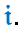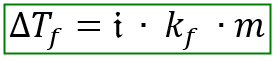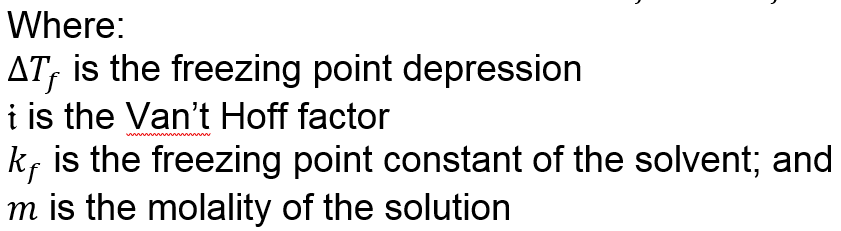# Problem: The freezing-point depression of a 0.091-m solution of CsCl is 0.320˚C. The freezing-point depression of a 0.091-m solution of CaCl2 is 0.440˚C. In which solution does ion association appear to be greater? Explain.

###### FREE Expert Solution

The problem asks us to find which solution has a greater ion association using freezing point depression data. We can determine which has a greater ion association indirectly from the  Van’t Hoff factorThe Van’t Hoff factor is the number of ions created once the compound dissociates. A higher Van't Hoff factor means greater dissociation and lesser association. Inversely, a lower Van't Hoff factor means greater ion association.

The freezing point depression equation is:92% (490 ratings)###### Problem Details

The freezing-point depression of a 0.091-m solution of CsCl is 0.320˚C. The freezing-point depression of a 0.091-m solution of CaCl2 is 0.440˚C. In which solution does ion association appear to be greater? Explain.